###### How to Solve Word Problems Programmatically

Chapter

12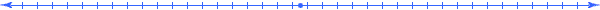Chapter 12.  Definition Models

 12.1 Basic Definitions 12.2 Sum (Total Seats) 12.3 Difference (of Rates) 12.4 Product (Man-Hours) 12.5 Quotient (Percent Increase) 12.6 Average (SAT Problem) 12.7 Area (College Algebra Problem) 12.8 Total Seats (Simultaneous Equations) 12.9 Escape Velocity (Advanced Physics) 12.10 Translating Phrases 12.11 Activities and Explorations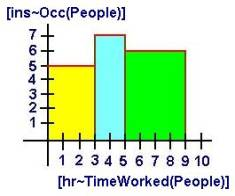12.1

#### Basic Dimension Definitions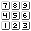Qty Value Meaning Relationship q1 x [kg~WeightAVG(Box1,Box2,Box3)] (q2+q3+q4)/3 q2 4 [kg~Weight(Box1)] q3 8 [kg~Weight(Box2)] q4 3 [kg~Weight(Box3)] q5

The fundamental operations of arithmetic (addition, subtraction, multiplication, and division) when applied to dimensions result in specific formulas as discussed in the previous chapter.   So the entry in the relationship column defines that operation.   In general, we consider an entry in the Relationship column as a way of defining dimensions in the Meaning Column.

For example the dimension "Average" invokes in our minds a formula, which in essence defines what we mean by "average".  This defining formula relates the quantity (with that meaning) to other quantities in the meaning table either previously defined or introduced further on in the table when the formula is introduced.  In this process, we focus our attention on the way that quantities relate to each other, and we formulate many small well-defined equations.  We suppress the desire to start solving these equations during the formulating process; this helps us to see the full model rather than just a glimpse of a particular state of the system.

Thus, the examples in this chapter often contain multiple equations containing multiple variables.  In later chapters, we will use algebra principles to solve such simultaneous equations.  For now (especially if you do not know how to solve such equations), just use a software application that solves systems of equation like the one at the site http://www.quickmath.com/ where you can select the menu option "Equation / Solve", and select the advanced version.  (See Chapter 15 for more information.)

12.2

#### Sum (Total)How many baseballs at a price of \$5.00 each can the team purchase if they raised a total of 150 dollars and already used some of that money to purchase 4 bats at a price of 30 dollar each?

Identify:

 Qty Value Meaning Relationship q1 x baseballs q2 5 [dol~Cost(Baseball)]/baseball q3 150 [dol~Raised] q4 4 bats q5 30 [dol~Cost(Bat)]/bat q6 [dol~Cost(Baseball)] q7 [dol~Cost(Bat)]

When identifying quantities in the problem, also look in previous meaning expressions for scaled dimensions not yet been defined and enter them on their own line.  Quantities q6 and q7 come from q2 and q5 respectively.

Relate:

 Qty Value Meaning Relationship q1 x baseballs q2 5 [dol~Cost(Baseball)]/baseball q6/q1 q3 150 [dol~Raised] q6+q7 q4 4 bats q5 30 [dol~Cost(Bat)]/bat q7/q4 q6 [dol~Cost(Baseball)] q7 [dol~Cost(Bat)]

Quantities q2 and q5 have proportional meaning expressions so we can create the corresponding ratio of quantities in the relationship column implying that q2=q6/q1 and q5=q7/q1.  In order to relate the meaning [dol~Raised] to any other meaning, we realize that the definition of the dimension Raised involves the total cost of the items; hence a sum in the Relationship column on the third line.

Formulate:

q2=q6/q1

q3=q6+q7

q5=q7/q1

or more specifically for this state of the system:

5=q6/x

150=q6+q7

30=q7/4

Solve:

q6=5*x  (from first equation)

q7=30*4=120  (from third equation)

150=5*x+120  (from second equation)

30=5*x

6=x

12.3

#### Difference (of Rates)A bus traveling 70 miles per hour made a trip in 4 hours.  How many minutes longer would it take if it traveled at 60 miles per hour? Difference of Rates

Source:  10 Real SATs, p. 145, The College Entrance Examination Board, New York

 Qty Value Meaning Relationship q1 x [min~Longer] q2 70 [mi~Length]/[hr~Time1] q7/q3 q3 4 [hr~Time1] q4 60 [mi~Length]/[hr~Time2] q7/q6 q5 60 [min~Longer]/[hr~Longer] x/q8 q6 [hr~Time2] q7 [mi~Length] q8 [hr~Longer] q6-q3

The first four quantities come from the word problem explicitly.  Notice that we only need to distinguish between two instances of a dimension like Time1 and Time2 if their values can differ.  In this situation, we do not need to distinguish between two different Length dimensions since this dimension is the same no matter what speed we use.  The next quantity provides a unit conversion between the two different units (minutes and hours) for the dimension "Longer".  The rest of the quantities finish documenting all the scaled dimensions used in the previous lines.

Obvious relationships come from the proportional meanings resulting in the divisions in the relationship column.  But a non-obvious relationship comes from the definition of the dimension "Longer".  This dimension invokes the generic dimension of difference, and in particular, the difference between Time2 and Time1 as indicated in the relationship column for q8.

Formulate:

q2=q7/q3

q4=q7/q6

60=x/q8

q8=q6-q3

More specifically for this state we have:

70=q7/4

60=q7/q6

60=x/q8

q8=q6-4

Solve:

q7=70*4=280  (from first equation)

q6=q7/60=280/60  (from second equation)

q8=280/60-4  (from fourth equation)

x=60*q8=60(280/60-4)=280-240=40

12.4

#### Product (Man-Hours)To do the project, 5 men worked during the first 3 hours, 7 men worked during the next 2 hours, and 6 men worked during the final 4 hours.  How many man-hours were spent on this project?Qty Value Meaning Relationship q1 x [ins~Occurrence(People)]*[hr~Time(People)] I h(x)dx q2

Formulate:

The information in the problem describes the horizon function that defines how the number of people working spreads out over the time dimension:

h(x) = 5 for 0<x<3

h(x) = 7 for 3<x<5

h(x) = 6 for 5<x<9

Solve:

x  = I h(x)dx = 5*3+7*2+6*4 = 53

By definition, the dimension man-hours represents the multiplication of the two dimensions "number of men (and women) working" and "number of hours worked".  More formally, we could translate this into a meaning expression such as:

[ins~Occurrence(People)]*[hr~Time(People)]

The area underneath the horizon function gives the result of this multiplication of two dimensions.  In this particular case, the definition reduces to the sum of products of each time period where a constant number of people worked times the number of people that worked during that time period.

12.5

#### Quotient (Percent Increase)The price of a sweater went up 20% since last year.  If last year’s price was 50 dollars, what is this year’s price? Difference of Rates

 Qty Value Meaning Relationship q1 x [dol~ThisYear] q2 20/100 [dol~Increase]/[dol~LastYear] q4/q3 q3 50 [dol~LastYear] q4 [dol~Increase] q1–q3

Formulate:

(20/100)=q4/50

q4=x-50

Solve:

We show in detail how to solve simultaneous equations in Chapter 15.  Briefly, in the first equation solve for q4=10 and substitute this number into the second equation (10=x-50) and solve to get x=60

Alternative Approaches:

There often exists many ways to express the same algebraic relationships and thus many ways in which we might enter information into the meaning table.  The end result is still the same; we still end up with all the values for each quantity q1, q2, ... in the meaning table.  In this case, instead of entering the relationship of q2, q4, q3 in the second row (for q2) we could have put it in the last row (for q4).  When entering more than one relationship into a row, we use an equal sign to connect it to the other relationships in the row.  (Remember that everything across the row equal each other.)

 Qty Value Meaning Relationship q1 x [dol~ThisYear] q2 20/100 [dol~Increase]/[dol~LastYear] q3 50 [dol~LastYear] q4 [dol~Increase] q1–q3 = q2*q3

Then we could have proceed just substituted the values for q1, q2, and q3 into the equation in the last row:

Formulate:

x-50=(20/100)*50

Solve:

x = (20/100)*50+50 = 10+50 = 60

12.6

#### Averages (SAT Problem)The average height of 4 members of a 6-person team is 175 cm.  What does the average height (cm) of the other 2 players have to be if the average height of the entire team equals 180 cm? Completing the Average

Source:  10 Real SATs, p. 144, The College Entrance Examination Board, New York

 Qty Value Meaning Relationship q1 x [cm~HeightAVG(PlayersGroup2)] q7 / q5 q2 4 [ins~Occ(PlayersGroup1)] q3 6 [ins~OccSUM(PlayersGroup1& PlayersGroup2)] q2 + q5 q4 175 [cm~HeightAVG (PlayersGroup1)] q8 / q2 q5 2 [ins~Occ(PlayersGroup2)] q6 180 [cm~HeightAVG (PlayersGroup1& PlayersGroup2)] q9 / q3 q7 [cm~HeightSUM(PlayersGroup2)] q8 [cm~HeightSUM (PlayersGroup1)] q9 [cm~HeightSUM (PlayersGroup1& PlayersGroup2)] q7 + q8

The definition of average height implies that we divide the total of a group's height by the number of players in that group.  This definition gives us the quotient expressions in the Relationship column.

Formulate:

x = q7 / 2

175 = q8 / 4

180 = q9 / 6 = (q7+q8) / 6

Solve:

q7 = 2*x

q8 = 175*4 = 700

180 = (q7+q8) / 6 = (2*x + 700) / 6

180*6 = 2*x + 700

1080 - 700 = 2*x

x = 380/2 = 190

12.7

#### Area (College Algebra Problem)A circular swimming pool, 20 feet in diameter, is enclosed by a wooden deck that is 3 feet wide.  What is the area of the deck?  How much fence is required to enclose the deck?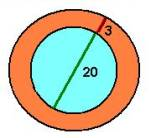Source:  College Algebra, Sullivan, p 27

 Qty Value Meaning Relationship q1 A [sqft~Area(Deck)] q5-q6 q2 P [ft~Circumference(LargeCircle)] p * q7 q3 20 [ft~Diameter(SmallCircle)] 2 * q8 q4 3 [ft~Width(Deck)] q9-q8 q5 [sqft~Area(LargerCircle)] p * q9^2 q6 [sqft~Area(SmallCircle)] p * q8^2 q7 [ft~Diameter(LargeCircle)] 2 * q9 q8 [ft~Radius(SmallCircle)] q9 [ft~Radius(LargeCircle)] q10 p=3.1416 [m~Circumference(Circle)]/[m~Diameter(Circle)]

Formulate:

A = q5-q6 = p*q9^2 - p*q8^2

P = p*2*q9

20 = 2*q8

3 = q9-q8

Solve:

q8 = 20/2 = 10   (equation 3)

q9 = 3 + q8 = 13   (equation 4)

A = 3.1416*(169-100) = 216.7

P = 3.1416*2*13 = 81.68

Even though the problem only asks for two quantities,  the meaning table provides values to all the related quantities considered when finding the requested quantities.  In general, the meaning table provides way of documenting the measurements of a system of things.

12.8

#### Total Seats (Simultaneous Equations)12.9

#### Escape Velocity (Advanced Physics)Find the minimum radius for a planet of mean density 5,500 kg/m3  and temperature 400 degrees Celsius which has retained oxygen in its atmosphere.

Source:  REA Super Review Physics, p.313

Find the minimum radius for a planet of mean density 5,500 kg/m3  and temperature 400 degrees Celsius which has retained oxygen in its atmosphere.

 Qty Value Meaning Relationship q1 r [m~Radius(Sphere)] q2 5500 [kg/m3~Density(Sphere)] q4 / q5 q3 400+273 [degK~Temperature(Sphere)] M/(3*R)* q6^2 q4 [kg~Mass(Sphere)] q5 [m3~Volume(Sphere)] (4/3)*p* q1^3 q6 [m/s~AverageVelocity(OxygenMoleculesInSphere)] =[m/s~EscapeVelocity(OxygenMoleculesInSphere)] sqrt(2*G* q4/q1) q7 p=3.1416 [m~Cir(Circle)]/[m~Dia(Circle)] q8 R=8.315 [J/(mol*degK)~GasConstant] q9 M=3.2E-2 [kg/mol~MolecularMass(Oxygen)] q10 G=6.67E-11 [N*m^2/kg^2~GravitationalConstant]

Definition Relationships Used:

 v = sqrt(3*T*R/M) Root-mean-squared velocity of gas molecules (solve for T to get line q3) V= (4/3)*p*r^3 Volume of sphere v = sqrt(2GM/r) Escape velocity of object

Formulate:

General Model:

5500 = q4 / q5

q5=(4/3)*3.1416* r^3

673 = .032/(3*8.315)* q6^2

q6 = sqrt(2*6.67*10^-11* q4/r)

Again using the equation solving application at http://www.quickmath.com/  we have: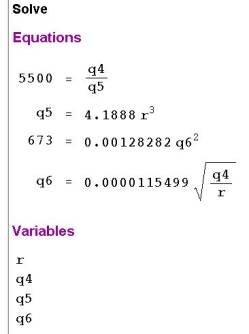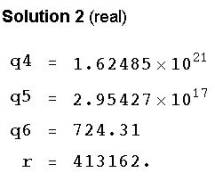Notice that we not only get the radius of the planet but also its mass (q4), density (q5), and the escape velocity (q6)

Notes:

The presentation in the REA book is typical of physics texts.  It mixes the “relate” steps with the “solve” steps and drags the reader through a path of proof like steps.  The unified math approach uses the meaning table and the 5 steps of modeling as follows:

First we identify 3 quantities, the desired radius r and two numeric constants given in the problem.  Then we assign meaning to each of these quantities.

We relate Temperature to dimensions Mass and Length through formulas in the Kinetic Theory of Gases.  Of the many equations available in that theory we choose the one that involves velocity because of the phrase “retain oxygen”.  What does “retain oxygen” mean?  The average speed of Oxygen molecules would need to be just under the Escape velocity.  So a minimum radius would be such that the escape velocity equals the temperature velocity, namely,  v=sqrt(2GM/r)

12.10

#### Translating PhrasesSum     Perimeter Difference Multiplication     Area     Volume     Force Division     Rate     Probability     Percent     Slope     Acceleration Average total, all, addition, combination boundary, edge, fence subtraction, decrease product, times, replicate region, field, expanse, surface capacity, lift, push, pull, squeeze, exertion quotient, fraction, ratio, rate per, chance, likelihood, odds proportion, rate, points gradient, incline, pitch speedup, quickening mean, approximate, estimate

Build lists of key phrases associated with a given dimension.  For example the above list provides some alternative words and/or phrases to dimensions that imply basic algebraic operations.

12.11

#### Activities and ExplorationsActivities:

 Practice Using the Meaning Table http://www.purplemath.com/modules/index.htm Use the meaning table to solve the problems found in the Word Problem Lessons (found toward end of  this webpage).  Compare not only your answers but the process you went through to get the answer.   Solve Definition Word Problems Use the meaning table to solve various problems that rely on definitions at sites like: http://www.edteach.com/algebra/problems/general_word_problems.htm http://www.columbiaview.net/LECTS/ALG/09.5_app-eqs.htm

Explorations:

 Explore Word Problems Using Definition of Interest http://www.purplemath.com/modules/investmt.htm

Exercises:

 Do Related Problems In Your Textbook

Copyright © 2004  Dr. Ranel E. Erickson